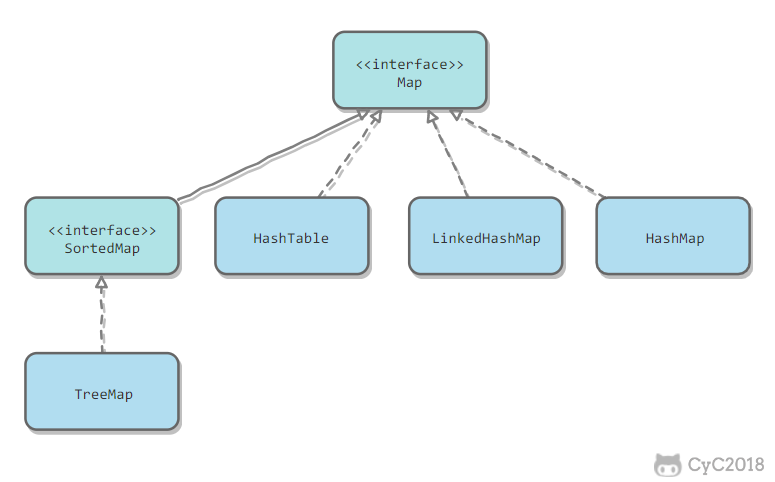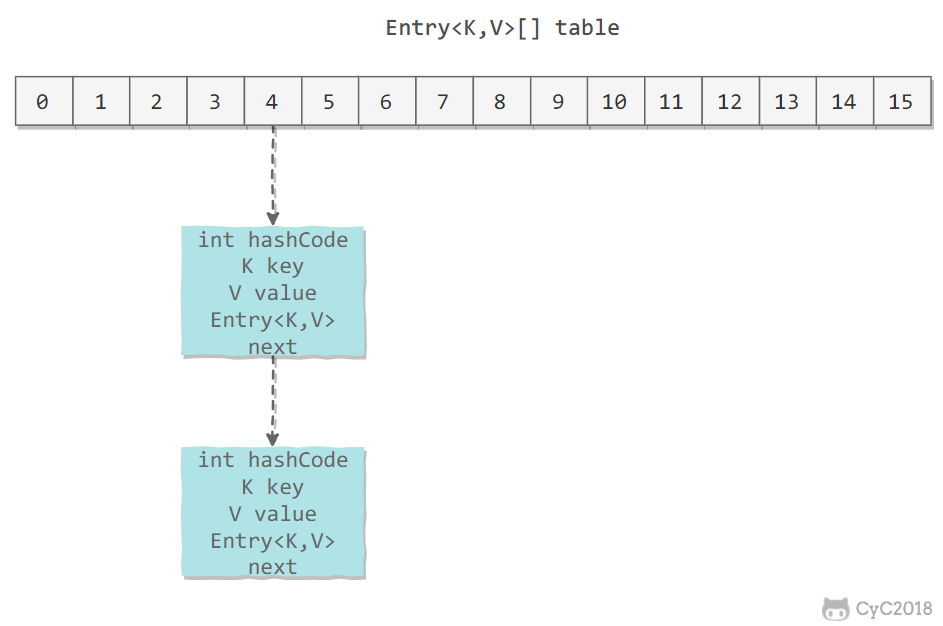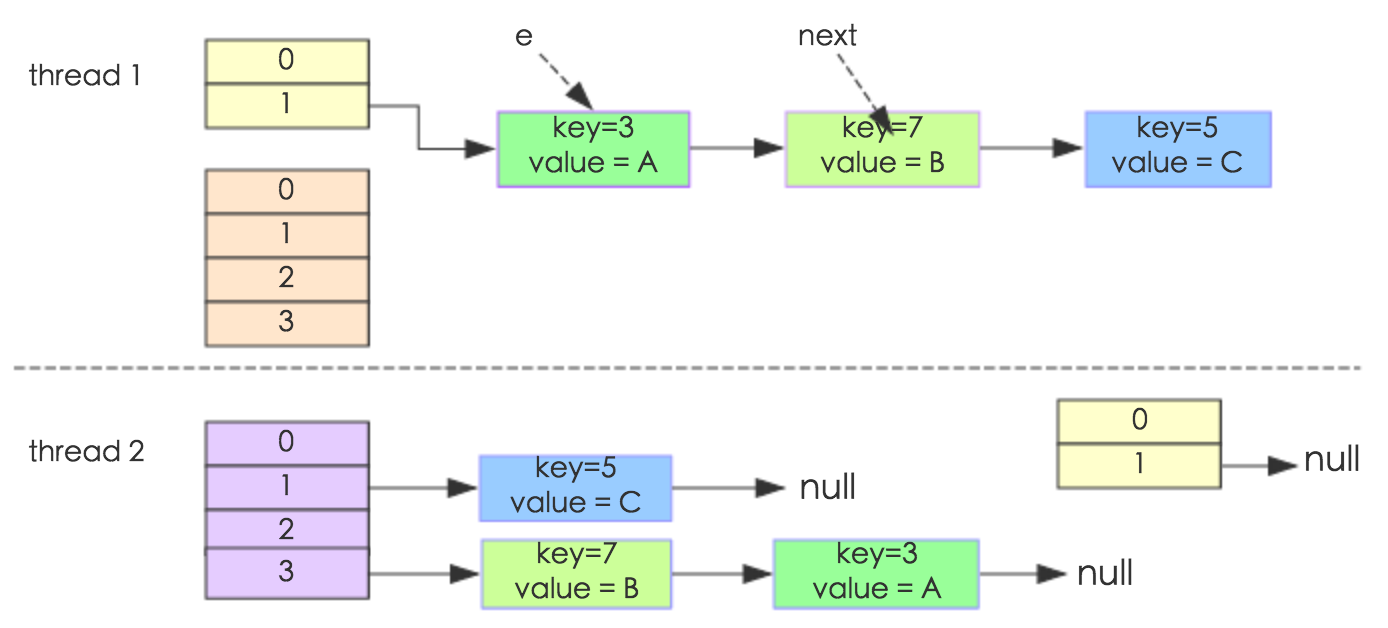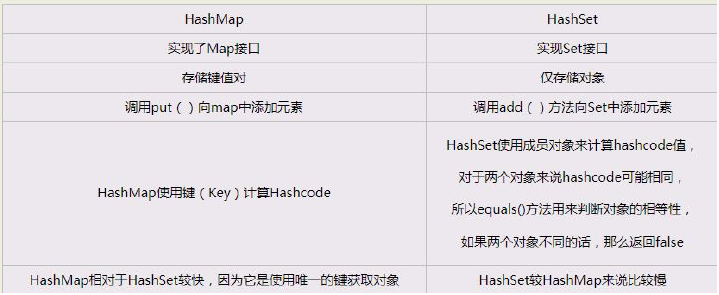# Java容器源码分析——HashMap

### HashMap

• TreeMap：基于红黑树实现;
• HashMap：基于哈希表实现;
• HashTable：和 HashMap 类似，但它是线程安全的，这意味着同一时刻多个线程可以同时写入 HashTable 并且不会导致数据不一致。它是遗留类，不应该去使用它。现在可以使用 ConcurrentHashMap 来支持线程安全，并且 ConcurrentHashMap 的效率会更高，因为 ConcurrentHashMap 引入了分段锁;# HashMap

## 1、存储结构

Entry 是构成哈希表的基石，是哈希表所存储的元素的具体形式内部包含了一个Entry类型的数组table;

Entry内部存储着键值对，包含了四个字段，从next字段可以看出Entry是一个链表;static class Node<K,V> implements Map.Entry<K,V> {
final int hash; // hash(key.hashCode())方法的返回值
final K key;//键值对的键
V value;//键值对的值
Node<K,V> next;//下一个节点

Node(int hash, K key, V value, Node<K,V> next) { //Node的构造函数
this.hash = hash;
this.key = key;
this.value = value;
this.next = next;
}

public final K getKey()        { return key; }
public final V getValue()      { return value; }
public final String toString() { return key + "=" + value; }

public final int hashCode() {
return Objects.hashCode(key) ^ Objects.hashCode(value);
}

public final V setValue(V newValue) {
V oldValue = value;
value = newValue;
return oldValue;
}

public final boolean equals(Object o) {
if (o == this)
return true;
if (o instanceof Map.Entry) {
Map.Entry<?,?> e = (Map.Entry<?,?>)o;
if (Objects.equals(key, e.getKey()) &&
Objects.equals(value, e.getValue()))
return true;
}
return false;
}
}


## 2、HashCode计算

static final int hash(Object key) {
int h;
return (key == null) ? 0 : (h = key.hashCode()) ^ (h >>> 16);
}


## 3、HashMap参数以及扩容机制

HashMap参数

static final int DEFAULT_INITIAL_CAPACITY = 1 << 4; //默认初始容量是16
static final int MAXIMUM_CAPACITY = 1 << 30;//最大容量是2的30次方
static final float DEFAULT_LOAD_FACTOR = 0.75f;//负载因子是0.75
static final int TREEIFY_THRESHOLD = 8;//这是一个阈值，当桶(bucket)上的链表数大于这个值时会转成红黑树
static final int UNTREEIFY_THRESHOLD = 6;//也是阈值同上一个相反，当桶(bucket)上的链表数小于这个值时树转链表
static final int MIN_TREEIFY_CAPACITY = 64;//树的最小的容量


void resize(int newCapacity) {
Entry[] oldTable = table;
int oldCapacity = oldTable.length;

// 若 oldCapacity 已达到最大值，直接将 threshold 设为 Integer.MAX_VALUE
if (oldCapacity == MAXIMUM_CAPACITY) {
threshold = Integer.MAX_VALUE;
return;             // 直接返回
}

// 否则，创建一个更大的数组
Entry[] newTable = new Entry[newCapacity];

//将每条Entry重新哈希到新的数组中
transfer(newTable);
table = newTable;
threshold = (int)(newCapacity * loadFactor);  // 重新设定 threshold
}



  void transfer(Entry[] newTable) {

// 将原数组 table 赋给数组 src
Entry[] src = table;
int newCapacity = newTable.length;

// 将数组 src 中的每条链重新添加到 newTable 中
for (int j = 0; j < src.length; j++) {
Entry<K,V> e = src[j];
if (e != null) {
src[j] = null;   // src 回收
// 将每条链的每个元素依次添加到 newTable 中相应的桶中
do {
Entry<K,V> next = e.next;
// e.hash指的是 hash(key.hashCode())的返回值;
// 计算在newTable中的位置，注意原来在同一条子链上的元素可能被分配到不同的子链
int i = indexFor(e.hash, newCapacity);
e.next = newTable[i];
newTable[i] = e;
e = next;
} while (e != null);
}
}
}


## 4、get源码

HashMap只需要根据key的哈希值定位到table数组的某个特定的桶，查找并返回该key对应的value即可；

以JDK1.7中的代码为例
public V get(Object key){
//若为null,调用getForNullKey方法返回对应的value;
if(key==null)
//从table的第一个桶中寻找key为null的映射；若不存在，直接返回null;
return getForNullKey();
//根据key的hashCode值重新计算它的hash码
int hash = hash(key.hashCode());
//找到table数组内对应的桶；
for(Entry<K,V> e = table[indexFor(hash,table.length)];
e!=null;
e = e.next;){
Object k;
//若搜索的key与查找的key相同，则返回相应的value
if(e.hash==hash&&((k==e.key)==key||key.equals(k)))
return e.value;
}
return null;
}
//针对键值为NULL的键值对
private V getForNullKey() {
// 键为NULL的键值对若存在，则必定在第一个桶中
for (Entry<K,V> e = table; e != null; e = e.next) {
if (e.key == null)
return e.value;
}
// 键为NULL的键值对若不存在，则直接返回 null
return null;
}


• 该key对应的值就是NULL;
• HashMap中不存在该key;

## 5、put源码

HashMap保存数据的过程，先判断key是否为null,若为null,则直接调用putForNullKey方法；若不为空，则先计算key的hash值，然后根据hash值搜索在table数组中的索引位置，如果table数组中该位置有元素，查找是否存在相同的key,若存在则覆盖原有key的value，否则将该元素保存在链表头部(最先保存的元素放在链表尾部)，若table此处没有元素，则直接保存；

public v put(K key,V value){
//当key==null时，调用putForNullKey,并将该键值保存到table上的第一个位置
if(key==null)
return putForNullKey(value);
//根据key的hashCode计算hash值
int hash = hash(key.hashCode());

//计算该键值对在数组中的存储位置(判断在哪个桶)
int i = indexFor(hash,table.length);

//在table的第i个桶上进行迭代，寻找key保存的位置
for(Entry<K,V> e = table[i];e!=null;e=e.next){
Object k;
//判断该条链表上是否存在hash值相同且key值相等的映射，若存在，直接覆盖value,并返回旧value;
if(e.hash==hash&&((k==e.key)==key||key.equals(k))){
V oldValue = e.value;
e.value = value;
e.recordAccess(this);
return oldValue;//返回旧值
}
}
modCount++;//修改次数+1，快速失败机制
//原HashMap中无该映射，将其添加至链表头部；
return null;
}

void addEntry(int hash,K key,V value,int bucketIndex){
//获取bucketIndex处的链表
Entry<K,V> e = table[bucketIndex];
//将新创建的 Entry 链入 bucketIndex处的链表的表头
table[bucketIndex] = new Entry<K,V>(hash,key,value,e);//参数e,是Entry.next;
//如果size超过threshold，扩充table大小，再散列
if(size++>=threshold)
resize(2*table.length);
}


HashMap采用了indexFor方法处理，简单高效。

static int indexFor(int h,int length){
return h&(length-1);//等价于取模运算
}


HashMap 中可以保存键为NULL的键值对，且该键值对是唯一的。若再次向其中添加键为NULL的键值对，将覆盖其原值。此外，如果HashMap中存在键为NULL的键值对，那么一定在第一个桶中

private v putForNullKey(V value){
//若key==null,则将其放入table的第一个桶内
for(Entry<K,V> e = table;e != null;e = e.next){
if(e.key == null){
//若已经存在key为null的键替换其值，并返回旧值
V oldValue = e.value;
e.value = value;
e.recordAccess(this);
return oldValue;
}
}
modCount++;
return null;
}


## 6、JDK 1.8中的优化(HashMap)

Java8中的改进

1. HashMap是数组+链表+红黑树；当链表长度>=8时转化为红黑树，利用红黑树快速增删改查的特点提高HashMap的性能；
2. Java8中对于hashMap的扩容不是重新计算所有元素在数组的位置，而是使用2次幂的扩展；元素要么在原位置，要么是在原位置再移动2次幂的位置；
3. HashMap在存放自定义类的时候,需要自定义类中的hashCode和equals,通过hash(hashCode)然后模运算，然后定位在Entry数组的下标，遍历之后的链表，通过equals比较有没有相同的key,有就直接覆盖，没有就重新创建一个Entry.

## 7、常见问题

1、HashMap为什么线程不安全？

HashMap在扩容的时候可能会生成环形链表，造成死循环；

2、HashMap中的key可以是任意对象或类型吗？

• 可以为null,但不能是可变对象，可变对象的属性改变，HashCode也会发生改变，导致无法查找到Map中的数据；
• 保证对于成员变量的改变能够使得对象的哈希值不变；

3、HashMap为什么可以插入null值

4、HashMap在高并发情况下会出现什么问题5、HashMap和HashSet之间的区别HashSet基于HashMap来实现，HashSet中存储的是一个对象，其中没有重复的元素
Hashset 底层是Hashmap 但是存储的是一个对象，Hashset 实际将该元素e 作为key 放入Hashmap,当key 值(该元素e)相同时，只是进行更新value，并不会新增加，所以set 中的元素不会进行改变。